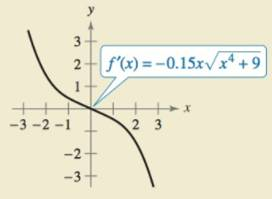Chapter 8.7, Problem 70E

Chapter
Section
Textbook Problem

HOW DO YOU SEE IT? Use the graph of f’ shown in the figure to answer the following.(a) Approximate the slope of f at x = − 1 . Explain.(b) Approximate any open intervals on which the graph of f is increasing and any open intervals on which it is decreasing. Explain.

(a)

To determine
The slope of function f at x=1.

Explanation

Given:

The graph of f(x):

Explanation:

As, f(x1) represents the slope of the function f(x) at x=x1 therefore, the slope of the function f(x) at

(b)

To determine
The open intervals on which the graph of f is increasing and decreasing.

Still sussing out bartleby?

Check out a sample textbook solution.

See a sample solution

The Solution to Your Study Problems

Bartleby provides explanations to thousands of textbook problems written by our experts, many with advanced degrees!

Get Started

Solve the equations in Exercises 126. x44x2=4

Finite Mathematics and Applied Calculus (MindTap Course List)

In problems 9-18, simplify the expressions with all exponents positive. 16.

Mathematical Applications for the Management, Life, and Social Sciences

Add or subtract as indicated. 90(3412)

Trigonometry (MindTap Course List)